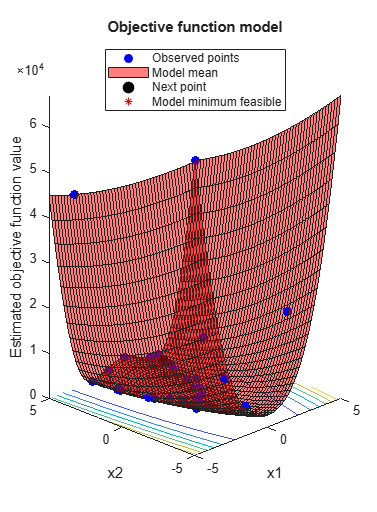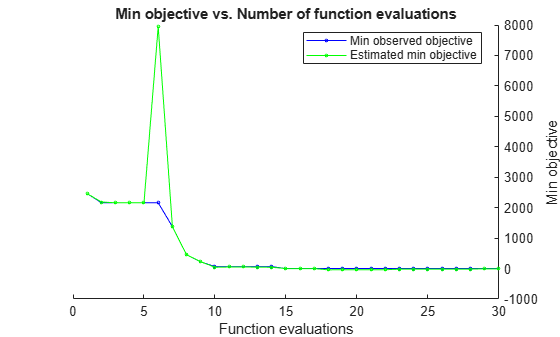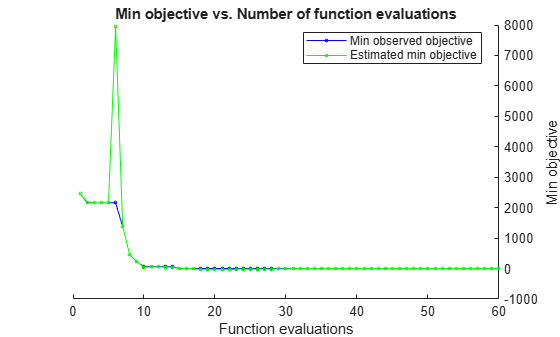# resume

Resume a Bayesian optimization

## Syntax

``newresults = resume(results,Name,Value)``

## Description

example

````newresults = resume(results,Name,Value)` resumes the optimization that produced `results` with additional options specified by one or more name-value arguments.```

## Examples

collapse all

This example shows how to resume a Bayesian optimization. The optimization is for a deterministic function known as Rosenbrock's function, which is a well-known test case for nonlinear optimization. The function has a global minimum value of `0` at the point `[1,1]`.

Create two real variables bounded by `-5` and `5`.

```x1 = optimizableVariable("x1",[-5,5]); x2 = optimizableVariable("x2",[-5,5]); vars = [x1,x2];```

Define an objective function named `rosenbrocks` that corresponds to Rosenbrock's function.

`type rosenbrocks.m % Display contents of rosenbrocks.m file`
```function f = rosenbrocks(x) f = 100*(x.x2 - x.x1^2)^2 + (1 - x.x1)^2; ```

Note: If you click the button located in the upper-right section of this page and open this example in MATLAB®, then MATLAB opens the example folder. This folder includes the objective function file.

Create a function handle for the objective function.

`fun = @rosenbrocks;`

For reproducibility, set the random seed, and set the acquisition function to `"expected-improvement-plus"` in the optimization.

```rng("default") results = bayesopt(fun,vars,"Verbose",0, ... "AcquisitionFunctionName","expected-improvement-plus");```View the best point found and the best modeled objective.

`results.XAtMinObjective`
```ans=1×2 table x1 x2 ______ ______ 1.7902 3.2287 ```
`results.MinEstimatedObjective`
```ans = -9.1194 ```

The best point is not very close to the optimum, and the function model is inaccurate. Resume the optimization for 30 more points (a total of 60 points), this time telling the optimizer that the objective function is deterministic.

```newresults = resume(results,"IsObjectiveDeterministic",true, ... "MaxObjectiveEvaluations",30);````newresults.XAtMinObjective`
```ans=1×2 table x1 x2 _______ _______ 0.96022 0.92508 ```
`newresults.MinEstimatedObjective`
```ans = -0.0094 ```

This time, the best point is closer to the true optimum, and the objective function model is closer to the true function.

## Input Arguments

collapse all

Bayesian optimization results, specified as a `BayesianOptimization` object.

### Name-Value Arguments

Specify optional pairs of arguments as `Name1=Value1,...,NameN=ValueN`, where `Name` is the argument name and `Value` is the corresponding value. Name-value arguments must appear after other arguments, but the order of the pairs does not matter.

Before R2021a, use commas to separate each name and value, and enclose `Name` in quotes.

Example: `resume(results,'MaxObjectiveEvaluations',60)`

You can use any name-value argument accepted by `bayesopt` except for those beginning with `Initial`. See the `bayesopt` Input Arguments.

Note

The `MaxObjectiveEvaluations` and `MaxTime` name-value arguments mean additional time or evaluations, above the numbers stored in `results`. So, for example, the default number of evaluations is `30` in addition to the original specification.

Additionally, you can use the following name-value argument.

Modify variable, specified as an `OptimizableVariable` object.

You can change only the following properties of a variable in an optimization.

• `Range` of real or integer variables. For example,

```xvar = optimizableVariable('x',[-10,10]); % Modify the range: xvar.Range = [1,5];```
• `Type` between `'integer'` and `'real'`. For example,

`xvar.Type = 'integer';`
• `Transform` of real or integer variables between `'log'` and `'none'`. For example,

`xvar.Transform = 'log';`

## Output Arguments

collapse all

Optimization results, returned as a `BayesianOptimization` object.

## Version History

Introduced in R2016b